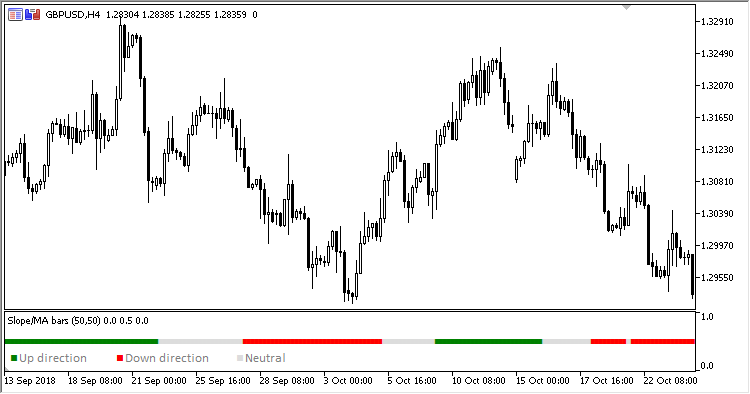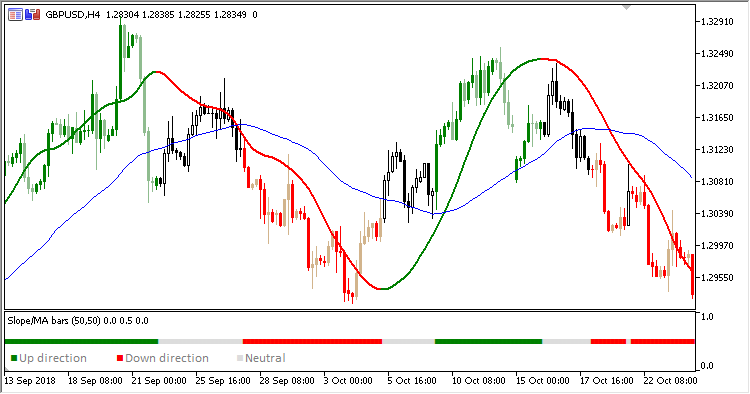Interesting script?
So post a link to it -
let others appraise it

You liked the script? Try it in the MetaTrader 5 terminal# Slope_Direction_Line_Bar - indicator for MetaTrader 5

Views:
1930
Rating:
Published:
2018.11.20 12:53

Trend-following Slope Direction Line / Moving Average confirmation bars signal indicator is an equivalent of Slope Direction Line / Moving Average confirmation candles indicator. It compares direction of two moving averages: Moving Average and Slope Direction Line and displays colored signal pointers in a separate window. Their colors correspond to the match/mismatch of Slope Direction Line and Moving Average lines direction.

It has six input parameters:

• MA period - Moving Average calculation period
• MA method - Moving Average calculation method
• MA applied price - Moving Average calculation price
• SDL period - Slope Direction Line calculation period
• SDL method - Slope Direction Line calculation method
• SDL applied price - Slope Direction Line calculation price
• If Moving Average and Slope Direction Line are directed upwards:
• the green signal pointer is set
• If Moving Average and Slope Direction Line are directed downwards:
• the red signal pointer is set
• In any other case, the gray signal pointer is placed.

Slope Direction Line indicator is not required - it is calculated by the indicator independently.Fig. 1. Slope Direction Line / Moving Average  confirmation barsFig. 2. Slope Direction Line / Moving Average  confirmation bars + Slope Direction Line / Moving Average confirmation candles

Translated from Russian by MetaQuotes Software Corp.
Original code: https://www.mql5.com/ru/code/22946VGridLine_Custom

The indicator plots one vertical line per day in a specified moment of timeColorRSI_X20

RSI_X20 indicator is able to color each line depending on its position relative to overbought and oversold areasSlope_MA_Confirmation

Slope Direction Line / Moving Average confirmation candles indicatorMoving Average applied price

Now you can test the indicator and select the price type from the ENUM_APPLIED_PRICE enumeration